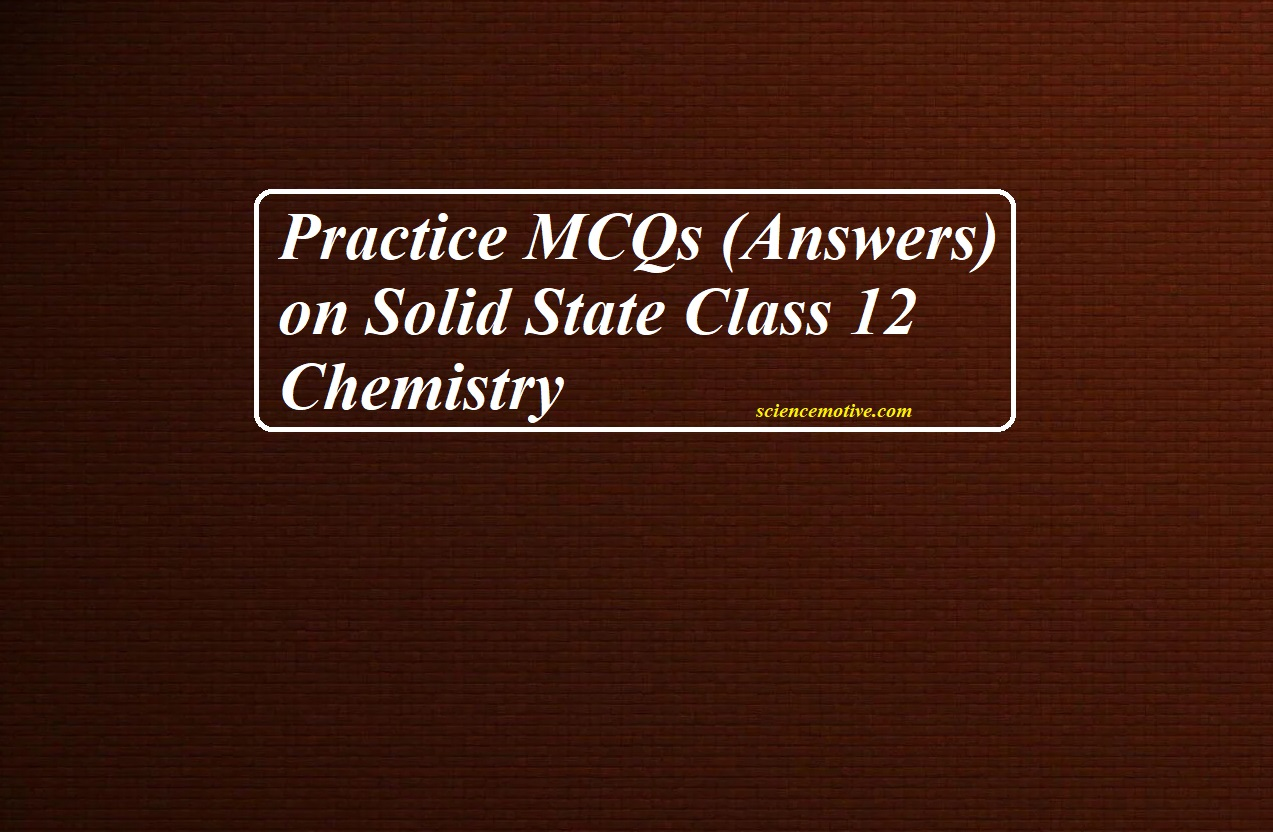# Practice MCQs (Answers) on Solid State Class 12 ChemistryTo Get Questions Click below
PRACTICE MCQS ON SOLID STATE CLASS 12 CHEMISTRY

Practice MCQs (Answers) on Solid State Class 12 Chemistry

Practice MCQs (Answers) on Solid State Class 12 Chemistry

1. (a) They have definite mass, volume, and shape

2. (c) Supercooled liquids

3. (d) Rubber

4. (d) Molecular crystal

5. (d) 1/8 part

6. (a) 32%

7. (b) 1

8. (d) 12

9. (c) 1/8, ½

10. (b) Edge centres and body centre

11. (b) X4Y5

12. (a) XY3

13. (c) P2Q

14. (b) Half

15. (b) fcc> bcc > simple cubic

16. (c) a=2r

17. (c) 14

18. (a) 52%

19. (b) ABCABC type

20. (d) 1

21. (b) bcc

22. (b) Tetrahedral void, octahedral void

23. (c) 9

24. (d) 4

25. (b) 10.5

26. (b) An equal number of cations and anions are missing

27. (c) The density of the crystal decreases

28. (a) Lattice sites containing electrons

29. (a) P gives rise to extra electrons while Al gives rise to holes

30. (c) Frenkel defect

31. (b) Ferromagnetic substances cannot be magnetized permanently

32. (a) all the domains get oriented in the direction of magnetic field.

33. (a) Frenkel defect

34. (a) Frenkel defect

35. (d) F-Centres

36. (b) AgBr

37. (c) Group 15

38. (c) it converts into paramagnetic substance

39. (b) Decrease

40. (b) Band Theory

41. (b) ZnO

42. (a) Vacancy defect

43. (d) SrCl2

44. (a) Assertion and reason both are correct statements and reason is a correct explanation for assertion.

45. (c) The assertion is a correct statement but the reason is the wrong statement.

46. (b) Schottky defects

47. (d) n-type semiconductor.

48. (c) √3 a/4: a/2√2

49. (c) 16лr3 /3

50. (b) 150

To Get Questions Click below
PRACTICE MCQS ON SOLID STATE CLASS 12 CHEMISTRY